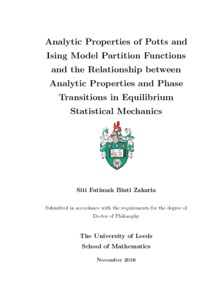# Analytic Properties of Potts and Ising Model Partition Functions and the Relationship between Analytic Properties and Phase Transitions in Equilibrium Statistical Mechanics

Zakaria, Siti Fatimah Binti (2016) Analytic Properties of Potts and Ising Model Partition Functions and the Relationship between Analytic Properties and Phase Transitions in Equilibrium Statistical Mechanics. PhD thesis, University of Leeds.Preview
Text
Zakaria_SFB_Mathematics_PhD_2016.pdf - Final eThesis - complete (pdf)
The Ising and $Q$-state Potts models are statistical mechanical models of spins interaction on crystal lattices. We study the partition functions on a range of lattices, particularly two- and three-dimensional cases. The study aims to investigate cooperative phenomena $-$ how higher level structure is affected by the detailed activity of a very large number of lower level structures. We investigate the analytic properties of the partition functions and their relationship to physical observables in equilibrium near phase transition. Our study is focussed on describing the partition function and the distribution of zeros of the partition function in the complex-temperature plane close to phase transitions. Here we first consider the solved case of the Ising model on square lattice as a benchmark for checking our method of computation and analysis. The partition function is computed using a transfer matrix approach and the zeros are found numerically by Newton-Raphson method. We extend the study of $Q$-state Potts models to a more general case called the $Z_Q$-symmetric model. We evidence the existence of multiple phase transitions for this model in case $Q=5,6,$ and discuss the possible connection to different stages of disordered state. Given sufficient and efficient coding and computing resources, we extend many previously studied cases to larger lattice sizes. Our analysis of zeros distribution close to phase transition point is based on a certain power law relation which leads to critical exponent of physical observable. We evidence for example, that our method can be used to give numerical estimates of the specific heat critical exponent $\alpha$.